# Curve toolbar

#####Catenary

Creates a curve that a hanging chain or cable assumes under its own weight when supported only at its ends.

#####Conic

Draw a conic section curve with options for the start, end, apex, and rho value.

#####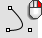Conic, Perpendicular

Draw a conic curve perpendicular at the start.

#####Conic, Tangent at start

Draw a conic curve tangent at the start.

#####Conic, Tangent at start and end

Draw a conic curve tangent at start and end.

#####Curve

Draw a curve from control point locations.

#####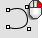CurveThroughPt

Fit a curve through point objects.

#####CurveThroughPolyline, Control point

Creates a control-point curve though polyline vertices.

#####CurveThroughPolyline, Interpolated

Creates an interpolated curve through polyline vertices.

#####HandleCurve

Draw chained Bézier curves with editing handles.

#####Helix

Draw a helical curve with options for number of turns, pitch, vertical, reverse, and around a curve.

#####Helix, Vertical

Draw a vertical helical curve.

#####Hyperbola

Draw a hyperbolic curve from focus points, vertices, or coefficient.

#####InterpCrv

Fit a curve through picked locations.

#####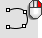HandleCurve

Draw chained Bézier curves with editing handles.

#####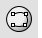InterpCrvOnSrf

Fit a curve through locations on a surface.

#####Parabola, Focus

Draw a parabolic curve from focus points.

#####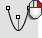Parabola, Vertex

Draw a parabolic curve from vertex and end.

#####Parabola3Pt

Draw a parabolic curve through three picked points.

#####Sketch

Drag the mouse to draw a curve.

#####Sketch, on surface

Drag the mouse to draw a curve on a selected surface.

#####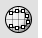Sketch, on mesh

Drag the mouse to draw a curve on a selected mesh object.

#####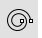Spiral

Draw a spiral curve with options for number of turns, pitch, flat, vertical, and around a curve.

#####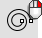Spiral, Flat

Draw a flat spiral curve.

#####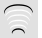TweenCurves

Create curves between two open or closed input curves.

Rhinoceros 7 © 2010-2021 Robert McNeel & Associates. 20-Oct-2021